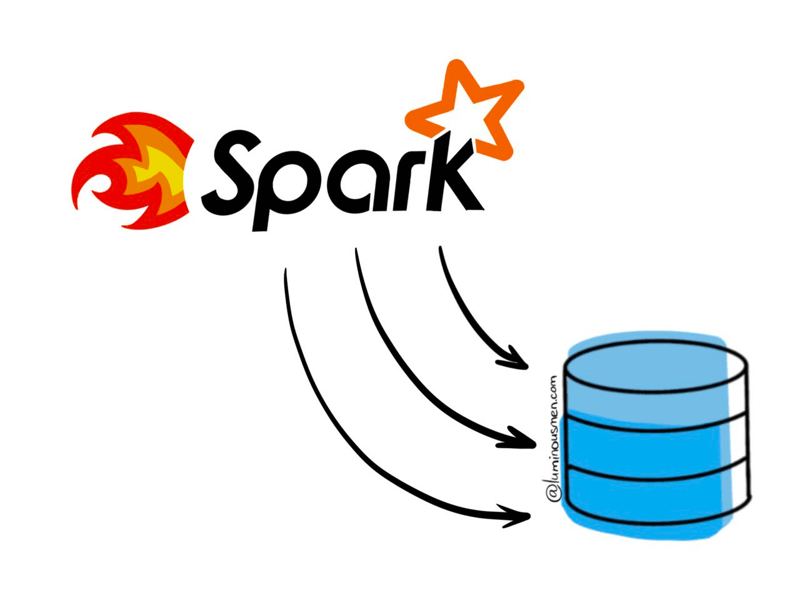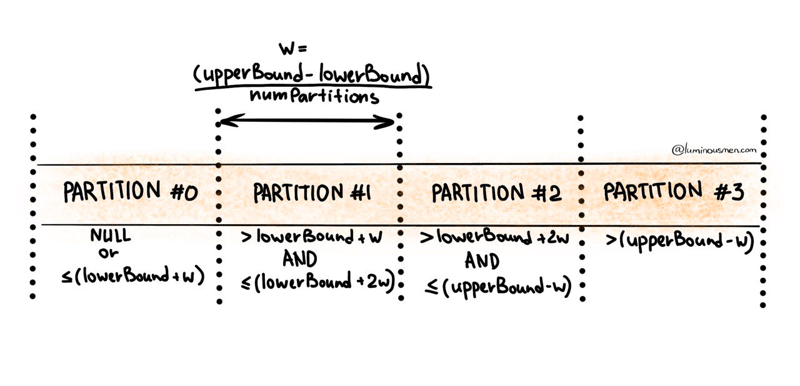In the context of the post we will be talking about reading from JDBC only but the same approaches applies to the writing as well.

On one of the projects I had to connect to SQL databases from Spark using JDBC. For those who do not know, JDBC is an application programming interface (API) to use SQL statements in, ahem, Java SE applications.

The example of usage from PySpark:

``````df = spark.read \
.format("jdbc") \
.option("url", "jdbc:postgresql:postgres") \
.option("dbtable", "db.table") \
.option("user", "user")\

df.write\
.format("parquet")\
.saveAsTable(...)
``````

It looks good, but it doesn't really work — it either works but very slowly, or it completely crashes depending on the size of the table.

When transferring large amounts of data between Spark and an external RDBMS by default JDBC data sources loads data sequentially using a single executor thread, which can significantly slow down your application performance, and potentially exhaust the resources of your source system.

In order to read data concurrently, the Spark JDBC data source must be configured with appropriate partitioning information so that it can issue multiple concurrent queries to the external database.

## Spark’s JDBC data source partitioning options

Spark JDBC reader is capable of reading data in parallel by splitting it into several partitions. There are four options provided by DataFrameReader:

• `partitionColumn` is the name of the column used for partitioning. An important condition is that the column must be numeric (integer or decimal), date or timestamp type. If the `partitionColumn` parameter is not specified, Spark will use a single executor and create one non-empty partition. Reading data will not be distributed or parallelized.
• `numPartitions` is the maximum number of partitions that can be used for simultaneous table reading and writing.
• The `lowerBound` and `upperBound` boundaries used to define the partition width. These boundaries determines how many rows from a given range of partition column values can be within a single partition.

To better understand what these are and what they control, let's go to the source code.

Apache Spark's implementation of partitioning can be found in this snippet of source code. From the source code we see that the data is partitioned using `partitionColumn`, which splits the values to the `numPartitions` groups using `stride` like this:### Example

For example, suppose you choose `numPartitions=10`, `lowerBound=0`, `upperBound=10000`. So the code would look something like this:

``````df = spark.read \
.format("jdbc") \
.option("url", "jdbc:postgresql:postgres") \
.option("dbtable", "db.table") \
.option("user", "user")\
.option("numPartitions", "10") \
.option("lowerBound", "0") \
.option("upperBound", "10000") \
``````

Under the hood, Spark generates an SQL query for each partition with an individual filter on the partitioning column. So for our example it is equivalent to running these 10 queries (one for each partition):

``````SELECT * FROM db.table WHERE partitionColumn <= 1000
SELECT * FROM db.table WHERE partitionColumn BETWEEN 1000 and 2000
...
SELECT * FROM db.table WHERE partitionColumn > 9000
``````

### Note on `lowerBound` and `upperBound`

The `lowerBound` and `upperBound` define partitioning boundaries, but they DO NOT participate in filtering rows of the table. Therefore, Spark partitions and returns ALL the rows of the table. It is important to note that all data will be read whether partitioning is used or not.

For example suppose we have `partitionColumn` data range in [0, 10000] and we set `numPartitions=10`, `lowerBound=4000` and `upperBound=5000`. As shown in the illustration above, the first and last partitions will contain all the data outside of the corresponding upper or lower boundary.

Another example, suppose we have `partitionColumn` data range in [2000, 4000] and we set `numPartitions=10`, `lowerBound=0` and `upperBound=10000`. In this case, then only 2 of the 10 queries (one for each partition) will do all the work, not ideal. In this scenario, the best configuration would be `numPartitions=10`, `lowerBound=2000`, `upperBound=4000`.

From these examples, we can conclude that lower and upper bounds should be close to the actual values present in the partitioning column. This can greatly affect performance. Probably the easiest way to determine them is something similar to this:

``````query = f"""SELECT MIN({partitionColumn}), MAX({partitionColumn}) FROM ({db.table})"""
.format("jdbc") \
.option("url", "jdbc:postgresql:postgres") \
.option("dbtable", "db.table") \
.option("user", "user")\
.option("query", query) \
lowerBound, upperBound = min_max_df.collect()
``````

## Optimizing performance

Performance issues can be checked either with the Spark UI or with your cluster metrics.

### Set `numPartitions` wisely

Spark tries to reuse existing partitions as much as possible and therefore indirectly the `numParitions` parameter also affects the degree of parallelism of all subsequent operations on the `DataFrame` until the `repartition` method is called. Thus, ideally, each of the executors should work with roughly the same amount of data so rows evenly distributed across partitions. In this way, we get properly balanced partitions, which helps improve application performance. We discussed that subject in this blog post.

Also keep in mind that by increasing number of partitions also increases the number of concurrent requests and connections made to the database. Most RDBMS systems have limits on the number of simultaneous connections. So try to keep the number of partitions at a reasonable limit. If you need a lot of concurrency after receiving JDBC rows (because you're running something CPU-bound in Spark), but you don't want to issue too many simultaneous database queries, consider using fewer partitions to read JDBC and then doing an explicit `repartition()` in Spark.

### Choose proper `partitionColumn`

The key to balanced partitions is to set the partitioning options correctly. With this in mind, we can optimize the whole process by choosing the right partition column and the `upperBound` and `lowerBound` values so that the `partitionColumn` strides are about the same size. To achieve this the values in `partitionColumn` should be evenly distributed to avoid skewing the data.

Analyze the columns available to determine if there are columns with high cardinality and even distribution that can be distributed among the desired number of partitions. These are good candidates for `partitionColumn`. Additionally, you should determine the exact range of values. Aggregations with different measures of centrality and skewness as well as histograms and basic key counts are good research tools. Depending on the RDBMS, you can use `width_bucket` (PostgreSQL, Oracle) or an equivalent function to get a decent idea of how the data will be distributed in Spark after loading with `partitionColumn`, `lowerBound`, `upperBound`, `numPartitons`.

``````SELECT
width_bucket(
partitionColum, lowerBound, upperBound, numPartitons
) AS bucket,
COUNT(*)
FROM db.table
ORDER BY bucket
``````

If possible, create a new one (perhaps a multi-column hash) to distribute the partitions more evenly.

### Consider index columns for `partitionColumn`

If you read using one of the partitioning options, Spark issues concurrent queries to the JDBC database. If these queries require a full table scan, this can cause bottlenecks in the database and become extremely slow. Partitioning is most efficient when performed on an indexed column. Therefore, when selecting a column for partitioning, you should consider the effect of indexes and choose a column so that queries of individual partitions can be run in parallel efficiently.

### Push down optimization

Spark can push down some conditions to the database, but only those in the `WHERE` clause. Everything else, such as constraints, counts, ordering, groups and conditions, is handled on the Spark side and requires both significant data transfer and handling with Spark, except `df.count()` — this operation simply goes into statistics which are stored in the database, in different situations join can also be pushed down.

This optimization reduces the amount of data loaded and helps you use query optimizations (such as RDBMS indexes) defined at the data source level.

You can prune columns and pushdown query predicates to the database with `DataFrame` methods, like this:

``````df.select("column1", "column2", "column3")
``````

### Use `batchsize` to boost writing speed

Another JDBC parameter is related to the record part and defines the number of batches performed for the insertion operation. This number is specified with the `batchsize` option. Spark will group the inserted strings into batches, each with the number of elements defined by the option.

``````df = spark.read \
.format("jdbc") \
.option("url", "jdbc:postgresql:postgres") \
.option("dbtable", "db.table") \
.option("user", "user")\
.option("batchsize","10000") \
``````

### Use `fetchsize` to boost reading speed

Yet another JDBC parameter which controls the number of rows fetched per iteration from a remote JDBC database. It defaults to low fetch size (e.g. Oracle with 10 rows).

``````df = spark.read \
.format("jdbc") \
.option("url", "jdbc:postgresql:postgres") \
.option("dbtable", "db.table") \
.option("user", "user")\
If you set `fetchsize` parameter too low, the workload can become queued up due to the large number of queries between Spark and the external database to get the full set of results. If `fetchsize` parameter is too high, it can lead to increased GC activity (so GC is suspended) and, in the worst case, OOM problems. The optimal parameter depends on the workload (as it depends on the result schema, the size of the rows in the results, and so on), but even a small increase in this parameter over the default value can result in huge performance gains.